# Module_3_1.pdf

30 May 2023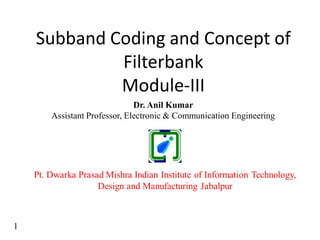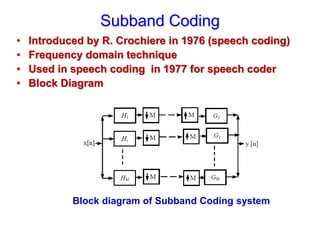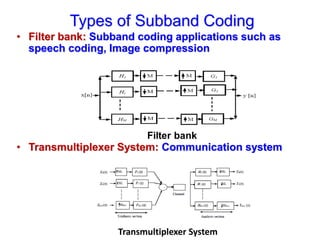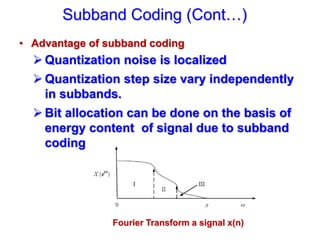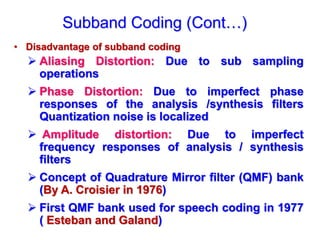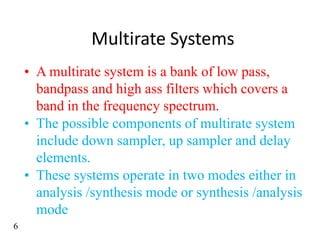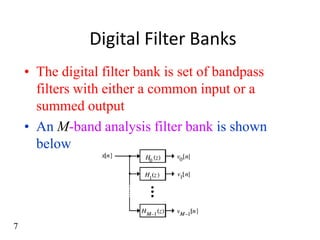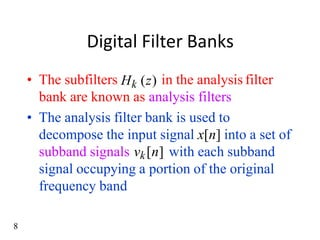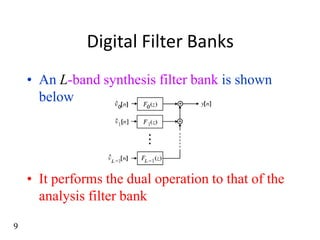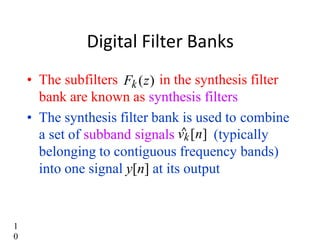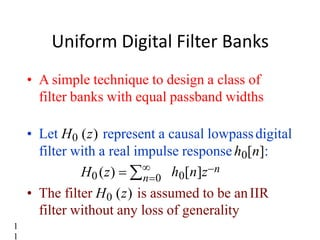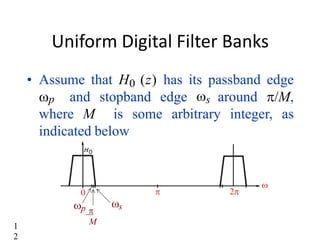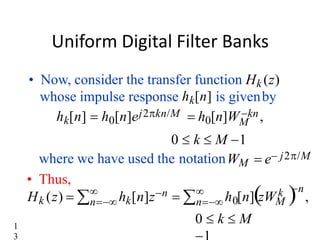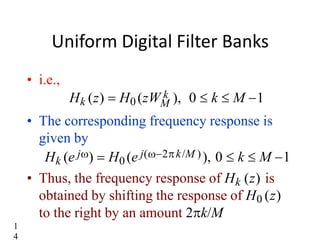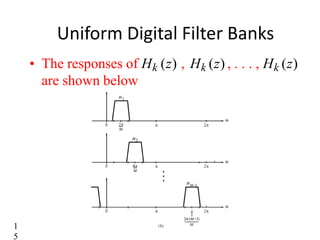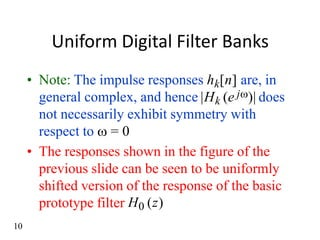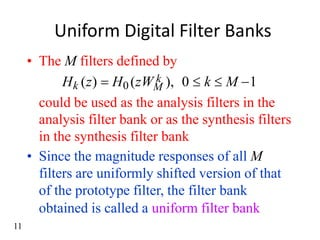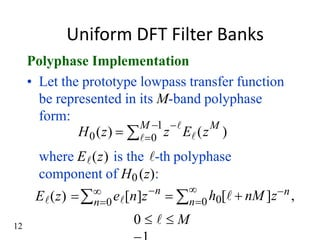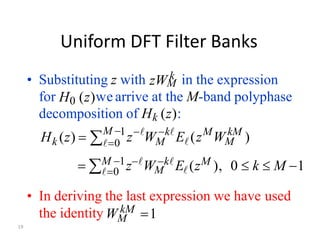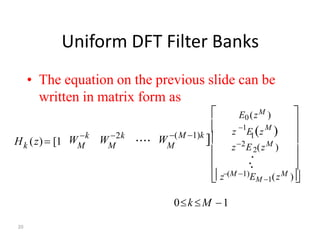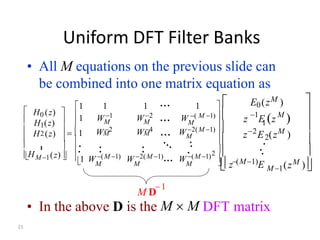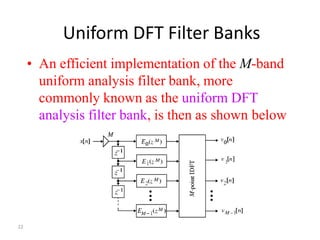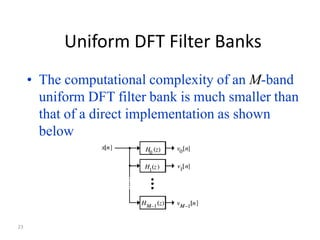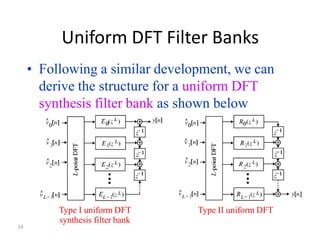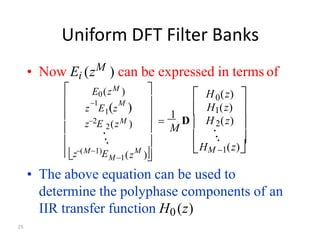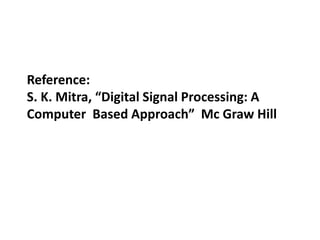1 sur 26

### Module_3_1.pdf

• 1. Subband Coding and Concept of Filterbank Module-III 1 Dr. Anil Kumar Assistant Professor, Electronic & Communication Engineering
• 2. • Introduced by R. Crochiere in 1976 (speech coding) • Frequency domain technique • Used in speech coding in 1977 for speech coder • Block Diagram Subband Coding Block diagram of Subband Coding system
• 3. • Filter bank: Subband coding applications such as speech coding, Image compression • Transmultiplexer System: Communication system Types of Subband Coding Transmultiplexer System
• 4. • Advantage of subband coding  Quantization noise is localized  Quantization step size vary independently in subbands.  Bit allocation can be done on the basis of energy content of signal due to subband coding. Subband Coding (Cont…) Fourier Transform a signal x(n)
• 5. • Disadvantage of subband coding  Aliasing Distortion: Due to sub sampling operations  Phase Distortion: Due to imperfect phase responses of the analysis /synthesis filters Quantization noise is localized  Amplitude distortion: Due to imperfect frequency responses of analysis / synthesis filters  Concept of Quadrature Mirror filter (QMF) bank (By A. Croisier in 1976)  First QMF bank used for speech coding in 1977 ( Esteban and Galand) Subband Coding (Cont…)
• 6. Multirate Systems • A multirate system is a bank of low pass, bandpass and high ass filters which covers a band in the frequency spectrum. • The possible components of multirate system include down sampler, up sampler and delay elements. • These systems operate in two modes either in analysis /synthesis mode or synthesis /analysis mode 6
• 7. Digital Filter Banks • The digital filter bank is set of bandpass filters with either a common input or a summed output • An M-band analysis filter bank is shown below 7
• 8. Digital Filter Banks 8 • The subfilters Hk (z) in the analysis filter bank are known as analysis filters • The analysis filter bank is used to decompose the input signal x[n] into a set of subband signals vk[n] with each subband signal occupying a portion of the original frequency band
• 9. Digital Filter Banks • An L-band synthesis filter bank is shown below • It performs the dual operation to that of the analysis filter bank 9
• 10. Digital Filter Banks 1 0 • The subfilters Fk (z) in the synthesis filter bank are known as synthesis filters • The synthesis filter bank is used to combine k belonging to contiguous frequency bands) into one signal y[n] at its output a set of subband signals v ^ [n] (typically
• 11. Uniform Digital Filter Banks 1 1 n0 h0[n]zn H0(z)   • A simple technique to design a class of filter banks with equal passband widths • Let H0 (z) represent a causal lowpassdigital filter with a real impulse responseh0[n]: • The filter H0 (z) is assumed to be anIIR filter without any loss of generality
• 12. Uniform Digital Filter Banks   0 2 s p  • Assume that H0 (z) has its passband edge p and stopband edge s around /M, where M is some arbitrary integer, as indicated below M 1 2
• 13. Uniform Digital Filter Banks 1 3 • Now, consider the transfer function Hk (z) whose impulse response hk[n] is givenby hk[n]  h0[n]ej2kn/M  h0[n]Wkn, M 0  k  M 1 where we have used the notationWM  e j2/M • Thus,   n k n Hk (z)  n hk[n]z  n h0[n]zWM  , 0  k  M
• 14. Uniform Digital Filter Banks 1 4 • i.e., Hk (z)  H0 (zW k ), 0  k  M 1 M • The corresponding frequency response is given by Hk (e j)  H0 (e j(2k/M )), 0  k  M 1 • Thus, the frequency response of Hk (z) is obtained by shifting the response of H0 (z) to the right by an amount 2k/M
• 15. Uniform Digital Filter Banks • The responses of Hk (z) , Hk (z) , . . . , Hk (z) are shown below 1 5
• 16. Uniform Digital Filter Banks 10 • Note: The impulse responses hk[n] are, in general complex, and hence |Hk (e j)| does not necessarily exhibit symmetry with respect to  = 0 • The responses shown in the figure of the previous slide can be seen to be uniformly shifted version of the response of the basic prototype filter H0 (z)
• 17. Uniform Digital Filter Banks obtained is called a uniform filter bank 11 • The M filters defined by M could be used as the analysis filters in the analysis filter bank or as the synthesis filters in the synthesis filter bank • Since the magnitude responses of all M filters are uniformly shifted version of that of the prototype filter, the filter bank Hk (z)  H0 (zW k ), 0  k  M 1
• 18. 12 Uniform DFT Filter Banks Polyphase Implementation • Let the prototype lowpass transfer function be represented in its M-band polyphase form:  0  M 1  M 0 z E (z ) H (z)    n0 n0 n h0[  nM ]zn, e[n]z  E(z)  where E(z) is the -th polyphase component of H0 (z): 0    M
• 19. Uniform DFT Filter Banks 19 • In deriving the last expression we have used • Substituting z with zW k in the expression  M k z W 0  M 1  k M kM E (z W ) H (z)  M for H0 (z)we arrive at the M-band polyphase decomposition of Hk (z): M  0 M   M 1zW kE (zM ), 0  k  M 1 M the identity W kM 1
• 20. Uniform DFT Filter Banks 20 • The equation on the previous slide can be written in matrix form as .... M M M k W k W 2k H (z) [1        W (M 1)k ]   z(M 1)EM 1(zM ) 2 M 1 z 1 E (z M ) E0(zM ) 0k M 1 z E .2(z ) ..
• 21. Uniform DFT Filter Banks 21 • All M equations on the previous slide can be combined into one matrix equation as M D  1 • In the above D is the M  M DFT matrix       (zM ) M 1 2 M 1 z 1 E (z M ) E0(zM ) z E .2(z ) ..     z(M1)E            2   1 1 1 1 1 1) M M M M M M M 1 W 2(M  W4 W2 W ( M 1)   W 1 W 2  (z) H (z)   1 H1(z) H0 (z)  M . . . M . . M . . . . ... ... ... 1 W (M 1) W 2(M 1)... W (M 1) ... 2 .  . H
• 22. Uniform DFT Filter Banks 22 • An efficient implementation of the M-band uniform analysis filter bank, more commonly known as the uniform DFT analysis filter bank, is then as shown below
• 23. Uniform DFT Filter Banks 23 • The computational complexity of an M-band uniform DFT filter bank is much smaller than that of a direct implementation as shown below
• 24. Uniform DFT Filter Banks 24 • Following a similar development, we can derive the structure for a uniform DFT synthesis filter bank as shown below Type I uniform DFT synthesis filter bank Type II uniform DFT
• 25. Uniform DFT Filter Banks 25 IIR transfer function H0(z) • The above equation can be used to determine the polyphase components of an           M (z )  M 1 z(M1)E z E1(z ) 1 M E0(zM ) 1      H (z)  HM 1(z) H1(z) 0   M D  H .2(z)  .. z2E .2(zM ) .. • Now Ei (zM ) can be expressed in terms of
• 26. Reference: S. K. Mitra, “Digital Signal Processing: A Computer Based Approach” Mc Graw Hill